# IT Go-Live Study

Gompertz Model for number of tickets from Go-Live to achieve some metrics, evaluate, compare with other projects and have some tools to create KPIs

At first, and looking some go-live tickets (issues) generated during some different Go-Lives I thought that classic sigmoid function could fit my model.

However, the real thing is that when you have release some new application in a production system, there is a gap between users starts to know/understand the functionality of the new tool and detect issues or have questions about how it works, etc.. So at the start of the go-live (if there are not really big issues, what is expected after a good UAT) you don't expect to have several issues, so the growth at very initial point is slower than some days after, and of course, when the new application/tool/functionality is coming more stable you should find that issues growth get slower another time.

This type of distribution is literally Gompertz function, so my expectation is that I could fit this function to go-live ticketing and use its parameters as metrics and a quick knowledge of the Go-Live and Post-GoLive achievements.

import numpy as np
import pandas as pd
import datetime
import sqlite3

#Gombertz fit and error discovery libraries
import sklearn.metrics as sklm
from scipy.optimize import curve_fit
from scipy.optimize import fsolve

#Graphics libraries
from plotnine import *


Our data is stored in SQLITE table, it comes from ticket managing tool is taking all tickets related to incidences, queries and small requests solved during Go-Live and post Go-Live support from a IT project.

con = sqlite3.connect("db.sqlite3")
cur = con.cursor()
sql = """SELECT count(requestid) as quantity,created_at as date
FROM Tool
WHERE groupme like '%Project Name%'
Other relevant filters from the company
GROUP BY created_at
"""
df = raw_data.copy()

raw_data.head() #Take a look of raw_data

index quantity date
0 13 2020-01-27
1 4 2020-01-28
2 8 2020-01-29
3 7 2020-01-30
4 2 2020-01-31

## Data analysis and exploration

### Basic data statistics

# Change data type of 'date' column to pandas datatime index type.
df['date'] = df['date'].astype('datetime64[ns]')
df.index = df['date'] #Set date as index to create time indexed dataframe.


Ok, we have now time series of our go-live project. Let's take a look to the raw data as it comes from our tool

all_tickets = (
ggplot(df,aes(x='date',y='quantity')) +
scale_x_date(date_breaks = "1 week",labels = date_format("%d-%b-%Y")) +
geom_line(color=orange, size=1.2) +
personal_theme +
labs(title='Tickets Opened during Go-Live Project',x='',y='')
)
all_tickets.draw();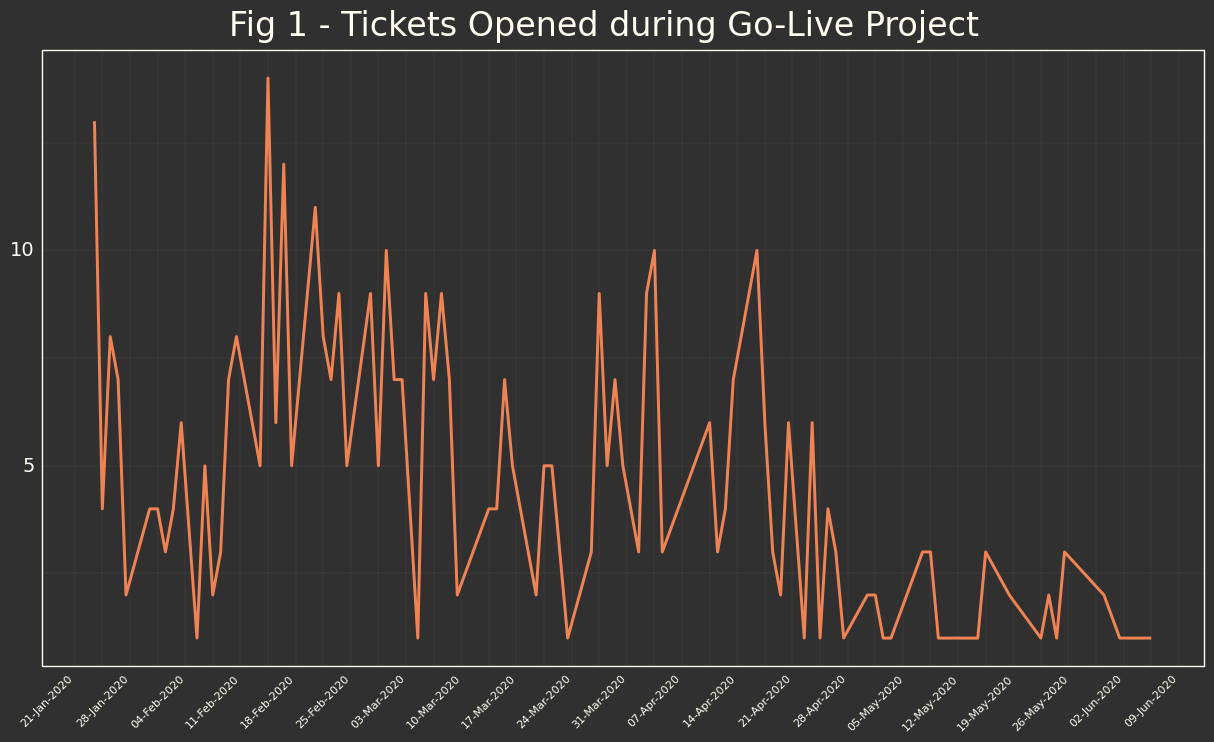We are going to take a look how it's distributed our tickets.

df['quantity'].describe()

count    88.000000
mean      4.772727
std       3.143054
min       1.000000
25%       2.000000
50%       4.000000
75%       7.000000
max      14.000000


Here, we have some different data that could help us to know or define additional metrics, but they are not really describing how the process of the go-live has worked, as it's not constant process and the behavior is different along the time.

Here the principal interesting information is that we are working over 88 days of tickets, which is good dataset for timeseries.

We could see distribution by month of the dataset, only to be much more familiar with it:

month_labels = ["Jan","Feb","Mar","Apr","May","Jun","Jul","Aug","Sep","Oct","Nov","Dec"]
month_distr = (
ggplot(df,aes(x='Month',y='quantity',group='Month')) +
scale_color_manual(values = personal_colors) +
scale_x_continuous(breaks=(1,2,3,4,5,6,7,8,9,10,11,12),labels=month_labels) +
geom_boxplot(color='red',fill=orange) +
personal_theme +
labs(title='Monthly Distribution',x='Month',y='Tickets Num.')
)
month_distr.draw()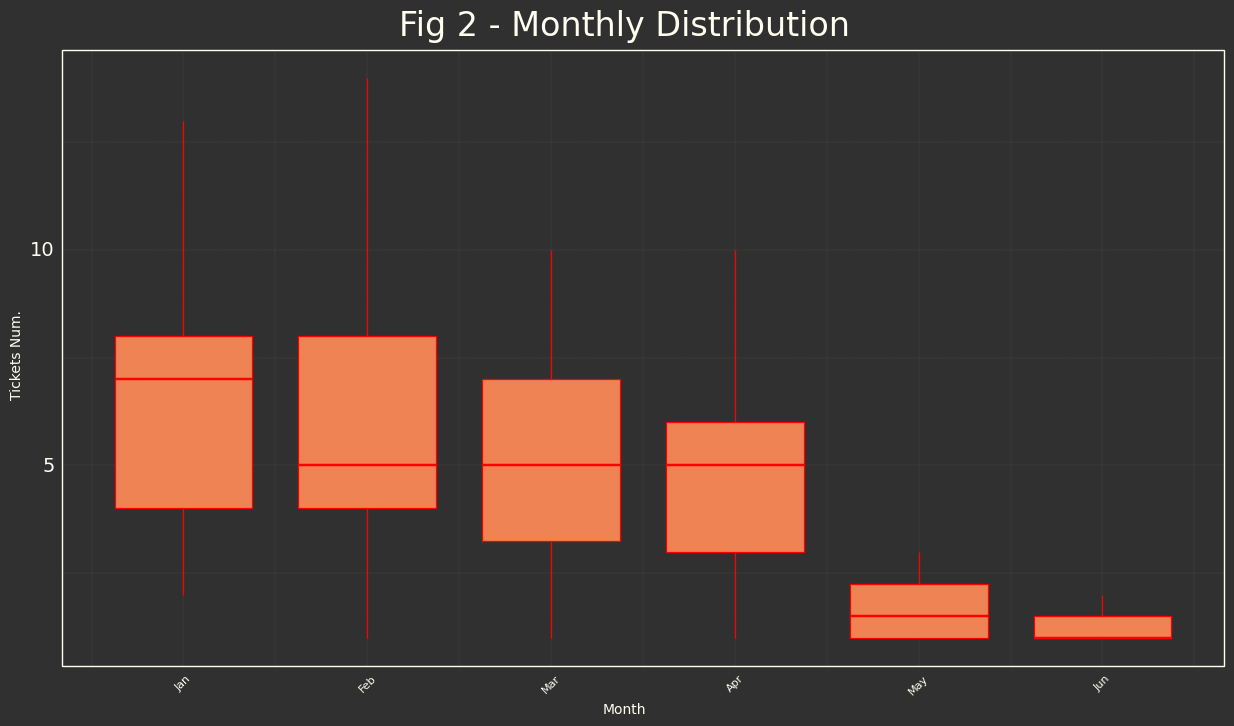Here we see that we have initial 2 month important volume of all generated tickets.

### Trending and Cumulative Data

Finally, the most important one, as this is the one that let us to see the real behavior of the go-live process in a general view, by analyzing its trend. I have applied 2 trend filters, rolling mean filter and Hodrick-Prescott for best smoothing reasons (because in this case it's not supposed to have seasonal component).

#Trend from filter Hodrick-Prescot
from statsmodels.tsa.filters.hp_filter import hpfilter
hpcycle, df['TrendHP'] = hpfilter(df.quantity)

df['trend14'] = df['quantity'].rolling(14).mean()
df_trend = pd.melt(df, id_vars='date', value_vars=['quantity','trend14','TrendHP'])
df_trend['variable'] = df_trend['variable'].replace({'quantity':'Raw Data','trend14':'Rolling 14d Mean','TrendHP':'Hodrick-Prescot'})


And graph

trend_graf = (
ggplot(df_trend,aes(x='date',y='value',color='variable')) +
scale_x_date(date_breaks = "1 week",labels = date_format("%d-%b-%Y")) +
scale_color_manual(values = personal_colors) +
geom_line() +
personal_theme +
labs(title='Raw Data and Trend Visualization',x='Weeks',y='Tickets Num.')
)
trend_graf.draw()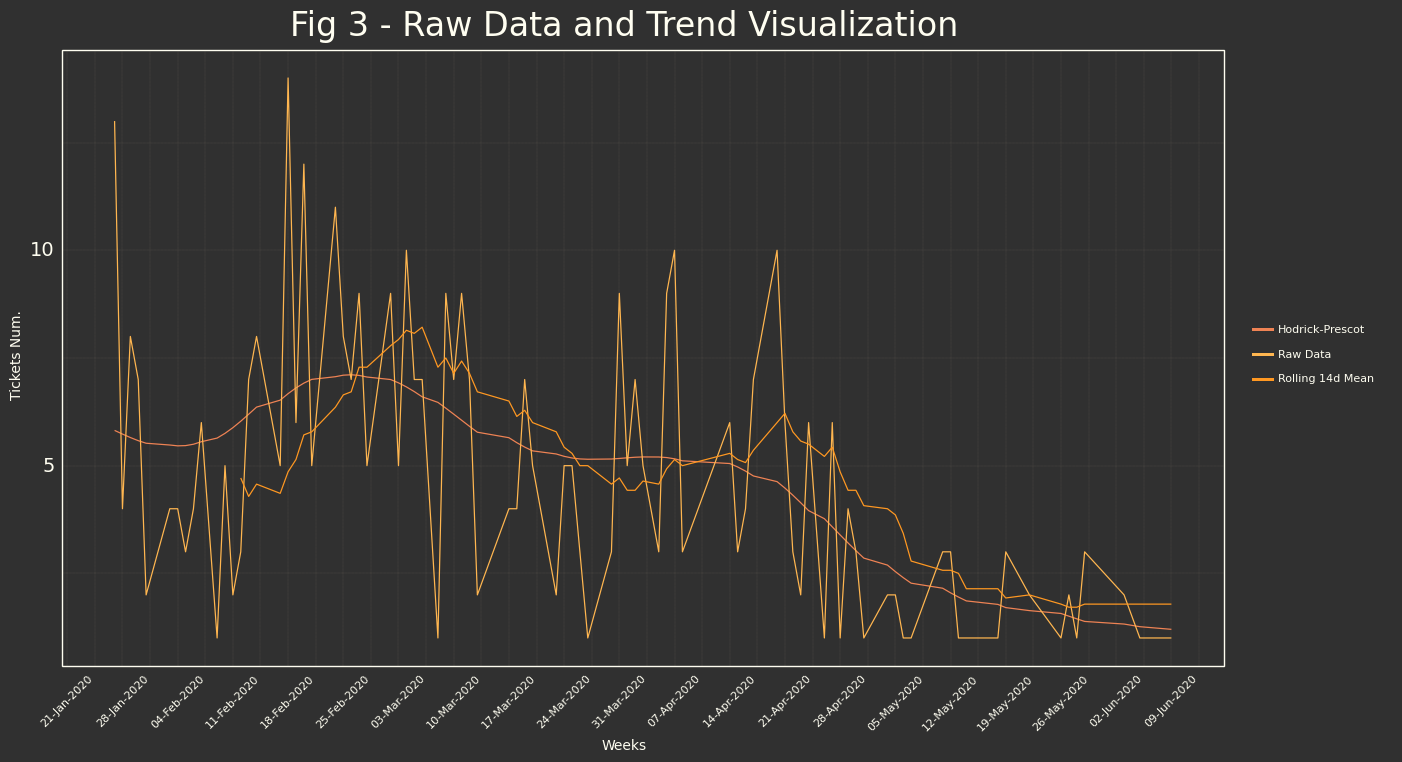And let me take the Trend individually, because it will let me explain better initial hypothesis.

trend_alone_graf = (
ggplot(df,aes(x='date',y='TrendHP')) +
scale_x_date(date_breaks = "1 week",labels = date_format("%d-%b-%Y")) +
scale_color_manual(values = personal_colors) +
geom_line(color=orange) +
personal_theme +
labs(title='Tickets Trend Smoothed',x='Weeks',y='Tickets Num.')
)
trend_alone_graf.draw()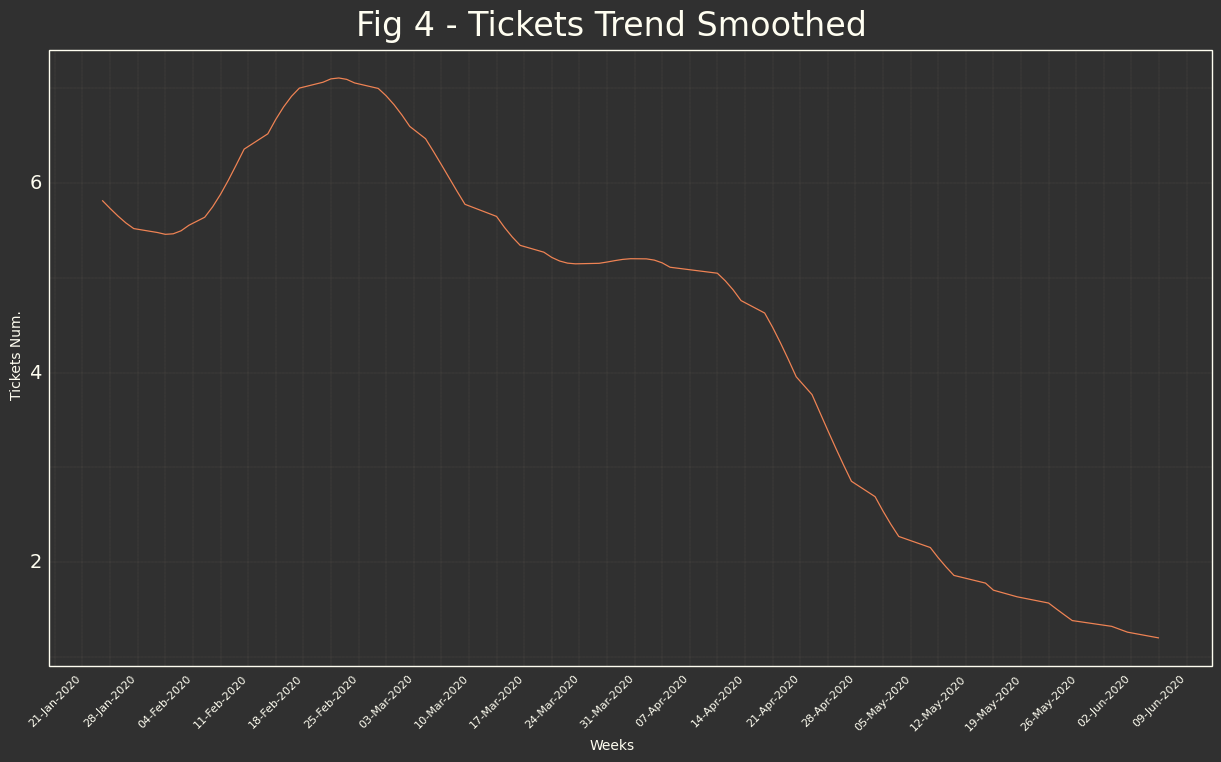This is smooth raw data, in the gompertz model it belongs to the 'derivate' function, we will need also the 'integration', it's basically the summatory area of the daily ticket function. We will then do cumulative summatory of daily tickets, the result should be the 'gompertz function' of our study case.

I need at first to have cumulative number of tickets:

df['CumSum'] = df['quantity'].cumsum()
#Melting data for ggplot
df_hipo = pd.melt(df, id_vars='date', value_vars=['TrendHP','CumSum'])
df_hipo['variable'] = df_hipo['variable'].replace({'TrendHP':'Trend','CumSum':'Cumulative'})


And we will graph it at the same time we re-catch our trend graph.

hipo_graf = (
ggplot(df_hipo,aes(x='date',y='value',color='variable')) +
scale_x_date(date_breaks = "1 week",labels = date_format("%d-%b-%Y")) +
scale_color_manual(values = personal_colors) +
geom_line() +
facet_wrap('variable',scales='free_y',nrow=2) +
personal_theme +
labs(title='Cumulative and Trend',x='Weeks',y='Tickets Num.')
)
hipo_graf.draw()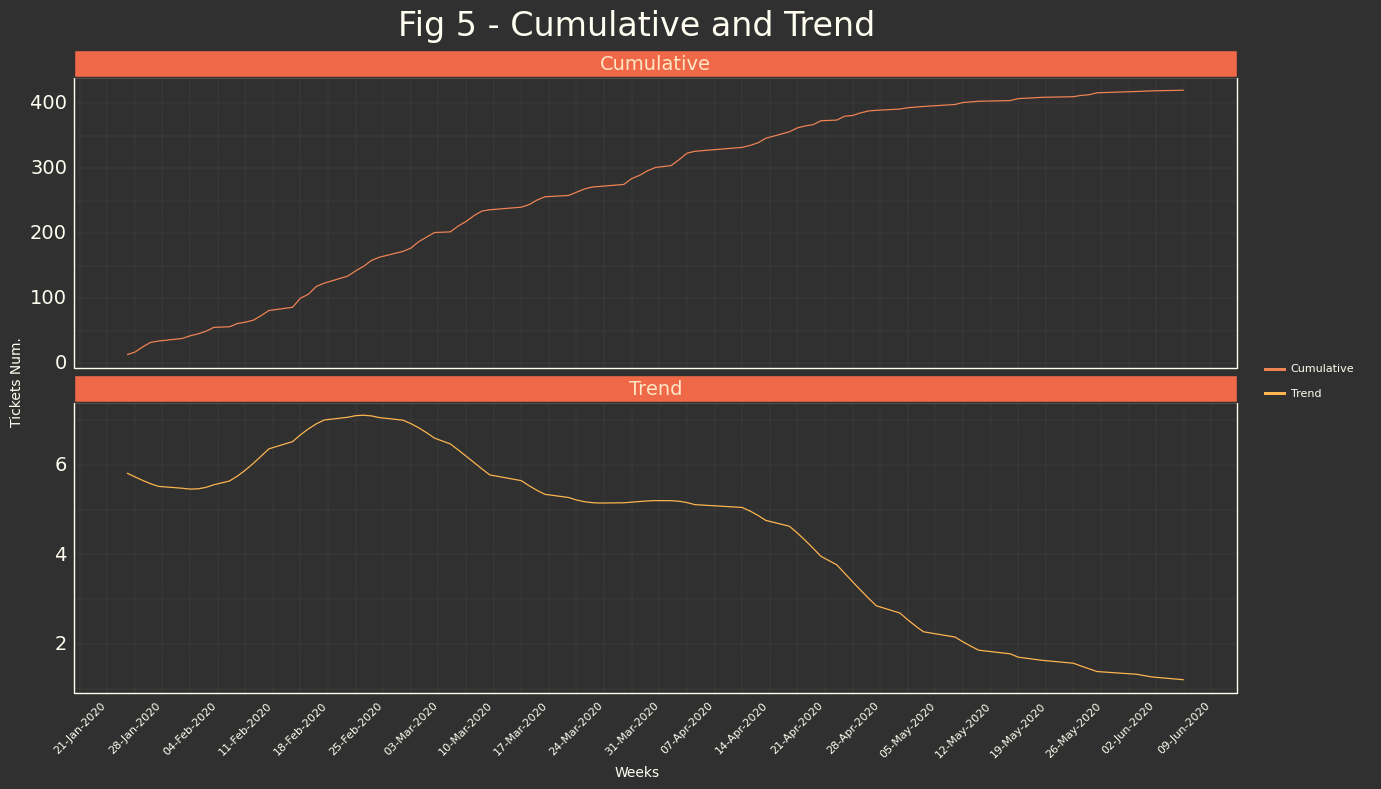## Hypothesis

### Little bit of Theory

Gopmertz function study is not something that belongs to disease world. There are some other studies that using this distribution has arrived to models that describe correctly its behavior. (for example vehicle saturation in a market).

Basically we need to take some assumptions:

1. Growth it's not symmetrical, slowest at the start and at the end.
2. Asymptotical decay when time -> $\infty$

Fig 6 - Here you can see this 2 assumptions described graphical way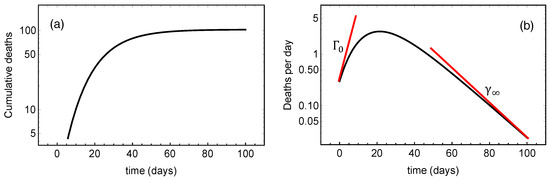These (Fig 6) could be described following these 2 functions:

And its derivate

#### Quick parameter description

• $a$ -> is an asymptote, in our case, total number of tickets created since Go-Live
• $b$ -> sets the displacement along x-axis, in our context could be useful to know when the major part of the users have started to test our new functionality, etc...
• $c$ -> sets the growth rate (y scaling), in terms of our context will give us the rate in how the tickets are created, it could be useful to know how much testing is done, if there are several users using the new functionality, etc..

### What we want?

We are comparing our time series trying to find some behavior that could remember us to gompertz function. I think that comparing Fig 6 with our exploratory dataset analysis Fig 5 from visual point of view we have solid reasons to think that could be good hypthoteical modelization to our time dataset.

Assumptions

1. In a Go-Live it's usual that big functionalities which are tested initially don't trigger any issue (if your UAT has been done correctly XD), so you expect to have slow growth at the start of go-live, and also you expect that after testing, solving issues etc.. at the end of the go-live you have also growth tending to 0. (In fact it's the way that you know that go-live has more or less finished).
2. For the same reason, you expect that an application that has been stabilized the issues decay asymptotically to 0.

### How?

We are going to fit Gompertz function that is non-linear equation using the same 'strategy' as it's used when fitting linear regression by least squares approximation.

SciPy provides function curve_fit() that will help us find the parameters of the gompertz function $(a,b,c)$ when variable is defined $x$.

## Modeling

### Creating functions

First of all we are going to create our functions (1) and (2) and check if we have created them well, with dummy data.

def gompertz_model(x,a,b,c):
return a*np.exp(-b*np.exp(-x/c))

def gompertz_derivate(x,a,b,c):
return (a*b*np.exp((-b*np.exp(-x/c))-x/c))/c

tt= np.linspace(0,100,100)

x_data = tt
y_data = gompertz_model(tt, 400, 50, 12)
y_data_d = gompertz_derivate(tt, 400, 50, 12)
#Melting for ggplot
dataset = pd.DataFrame({'Items': x_data, 'Gompertz Func.': y_data,'Gompertz Der.':y_data_d})
data_test = pd.melt(dataset, id_vars='Items', value_vars=['Gompertz Func.','Gompertz Der.'])


And graph them to check our known functions:

test_model = (
ggplot(data_test,aes(x='Items',y='value',color='variable')) +
scale_color_manual(values = personal_colors) +
geom_line() +
facet_wrap('variable',scales='free_y',nrow=2) +
theme_xkcd() +
theme_drawing +
labs(title='Fig 7 - Model Testing',x='Time',y='Tickets Num.')
)
test_model.draw()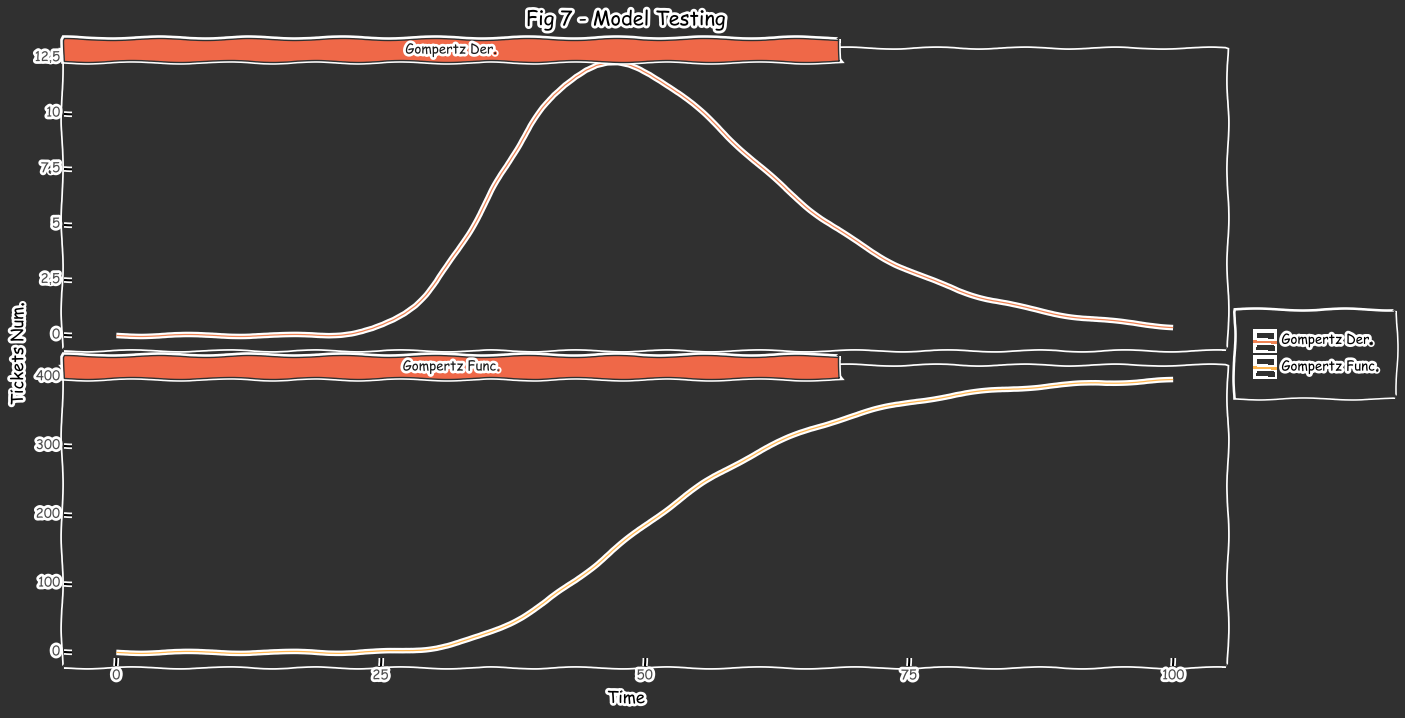Well, it seems that we have some type of sigmoid function running and I am quite sure that it is our particular case for gompertz sigmoid function :), please check Fig 5 also to check similarities.

Ok, it's the moment, we will use non-linear least squares regression to fit our function to our given data array (cumulative tickets by day).

## Fitting

First of all we will need to change 'date' variable to sequence and this will be our $x$.

df['DayCount'] = np.arange(1,df['quantity'].shape+1)


So let's construct our variables:

x = df['DayCount'].values
y = df['CumSum'].values

y0 = y
yf = y[-1]

print("--------------------------------")
print("Initial number of tickets: ", y0)
print("Current number of tickets: ", yf)
print("Number of days:            ", x[-1])

Some data for our adjustment:
--------------------------------
Initial number of tickets:  13
Current number of tickets:  420
Number of days:             88


Modeling

#Gompertz Modelization'
fit_i = curve_fit(gompertz_model,x,y)
ai,bi,ci = fit_i
sigma_ai, sigma_bi, sigma_ci = np.sqrt(np.diag(fit_i))

print('Final parameters for our modeled function:')
print('------------------------------------------')
print('a = {:.3f}'.format(ai),'+/- {:.3f}'.format(sigma_ai))
print('b = {:.3f}'.format(bi),'+/- {:.3f}'.format(sigma_bi))
print('c = {:.3f}'.format(ci),'+/- {:.3f}'.format(sigma_ci))

Final parameters for our modeled function:
------------------------------------------
a = 456.449 +/- 3.662
b = 3.186 +/- 0.051
c = 23.985 +/- 0.437


## Model Analysis

We have the model, now let's compare with our data, starting with Cumulative tickets over the time. In terms of units, we will continue working with 'days'.

#Creating new column using day as variable in our modelized function.
df['Model'] = df['DayCount'].apply(lambda x:gompertz_model(x,ai,bi,ci))
#ggplot melting
df_model = pd.DataFrame({'Days': df['DayCount'], 'Real Data':df['CumSum'],'Gompertz Model':df['Model']})
df_model = pd.melt(df_model, id_vars='Days', value_vars=['Real Data','Gompertz Model'])

model_graf = (
ggplot(df_model,aes(x='Days',y='value',color='variable')) +
scale_color_manual(values = personal_colors) +
geom_line() +
personal_theme +
labs(title='Fig 8 - Real Data vs Modeled Data',x='Days from Go-Live Start',y='Tickets Num.')
)
model_graf.draw()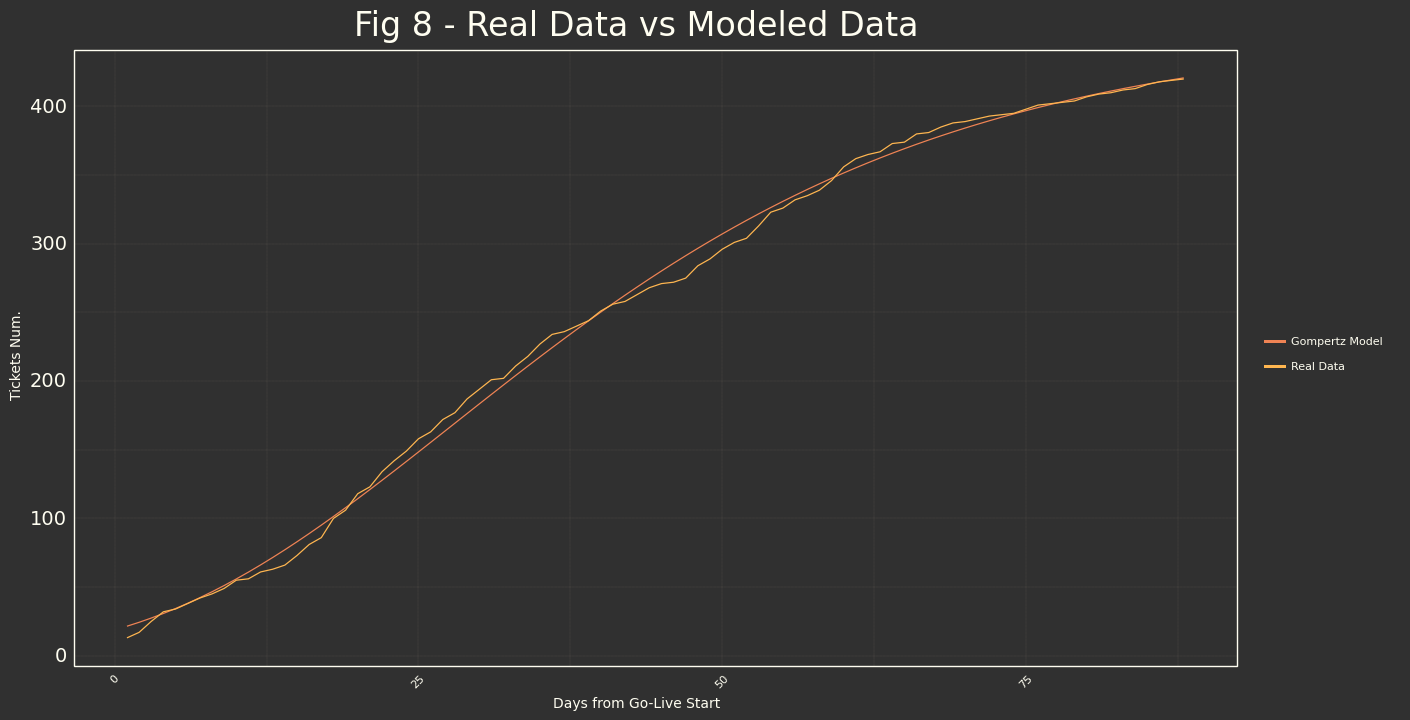Well it seems good.

Let's check derivate function (in the real life, our smooth filtered real tickets creation), we are going to do the same as before, we are going to apply our fitted parameters, with derivate function, apply to ordered days and compare with raw_data and filtered trend.

df['ModelD'] = df['DayCount'].apply(lambda x:gompertz_derivate(x,ai,bi,ci))
#Melting for ggplot
df_modelD = pd.DataFrame({'Days': df['DayCount'], 'Real Data':df['quantity'],'Smooth HP':df['TrendHP'],'Gompertz Model':df['ModelD']})
df_modelD = pd.melt(df_modelD, id_vars='Days', value_vars=['Real Data','Gompertz Model','Smooth HP'])

modeld_graf = (
ggplot(df_modelD,aes(x='Days',y='value',color='variable')) +
scale_color_manual(values = personal_colors) +
geom_line() +
personal_theme +
labs(title='Fig 9 - Real Data/Smooth vs Modeled Data',x='Days from Go-Live Start',y='Tickets Num.')
)
modeld_graf.draw()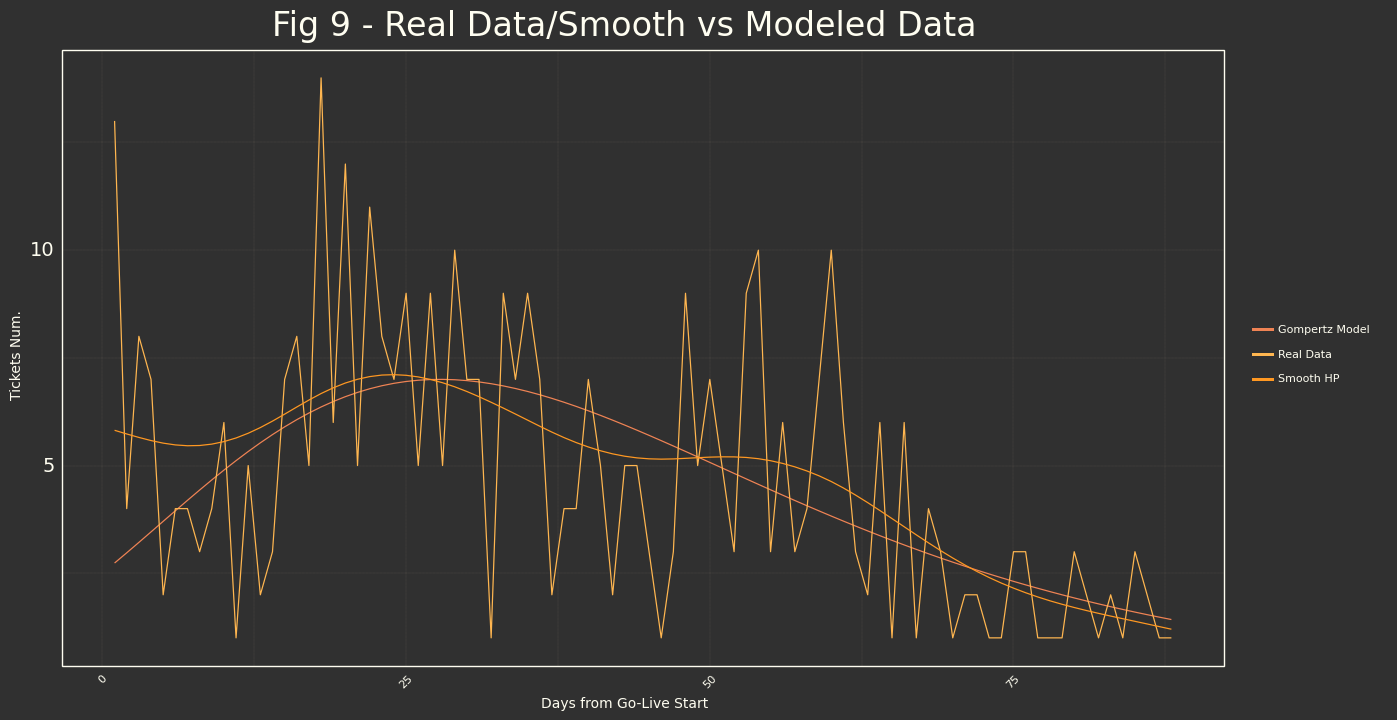I think that it's quite beautiful the way as the model fits to the real data. We are going to evaluate it with objective data, but in terms of visualization I think that Gompertz could be good way to evaluate how a project is released in production system and how confidence, stable and well tested it is, by comparing Gompertz function parameters, with objectives, or other projects you have analyzed.

### Model Accuracy and Some other possible data

Note: We will need to found roots for $y''$ so, we are going to develop it a bit

We have our derivate:

(2) $y'(x) = \frac{abe^{-be^{-x/c}-x/c}}{c}$

And we are going to derivate another time: (3) $y''(x) = - \frac{ab(e^{x/c}-b)e^{-be^{-x/c}-2x/c}}{c^2}$

def gompertz_derivate2(x,a,b,c):
numerator = a*b*(np.exp(x/c)-b)*np.exp((-b*np.exp(-x/c))-2*x/c)
return -(numerator/(c*c))


We are going to calculate our accuracy through scikit-learn statistical error/accuracy functions. And also we will try to find the 'maximum' of gompertz function, as it's also relevant data to find and to compare.

x = df['DayCount'].values
y = df['CumSum'].values

y_pred = gompertz_model(x,ai,bi,ci)

MSLE=sklm.mean_squared_log_error(y,y_pred)
MSE =sklm.mean_squared_error(y,y_pred)
print("Mean squared log error (MSLE): ", '{:.3f}'.format(MSLE))
print("Root Mean squared error (RMSE): ", '{:.3f}'.format(np.sqrt(MSE)))
R2 = sklm.r2_score(y,y_pred)
print("R2 score: ", '{:.3f}'.format(R2))

raw_sol = fsolve(lambda x : gompertz_derivate2(x,ai,bi,ci), 40)
#40 is an estimation by looking graph
#(20-40 any number is fine, as our maximum is there and we need for solve non-linear function)
#Check the zero.
check_value = gompertz_derivate2(raw_sol,ai,bi,ci)
print('How zero is our solution?',check_value)
sol = int(round(raw_sol))
print('Days to the Maximum since Go-Live: ', sol)
datesol = datetime.datetime.strftime(df.index + datetime.timedelta(days=sol), ' %d, %b %Y' )
print('Day of flattening of Post Go-Live Support: ',datesol)

Mean squared log error (MSLE):  0.006
Root Mean squared error (RMSE):  6.544
R2 score:  0.998
How zero is our solution? -4.0681485437308383e-17
Days to the Maximum since Go-Live:  28
Day of flattening of Post Go-Live Support:   24, Feb 2020


MSLE: Mean squared logarithmic error (MSLE) can be interpreted as a measure of the ratio between the true and predicted values, will treat small differences between small true and predicted values approximately the same as big differences between large true and predicted values. Finally, MSLE also penalizes underestimates more than overestimates, introducing an asymmetry in the error curve.

In our case is fine, but it's not really significant as we have low values...

RMSE: RMSE is the average squared difference between the estimated values and its root (the good thing is that it comes to us in the same units so it's in accumulated tickets/day and it's really good. $RMSE = \sqrt{\frac{1}{n}\sum^n_{i=1}(Y_i - Y_i)²}$

$R^2$: The great one, $R^2$ near to 1 means that we are really close to perfect model fit of our raw data behavior, and we have achieve $R^2=$ close to 1, it's not a surprise as we have seen in previous visualization that something has to be really wrong to don't have number near to 1.

Finally some additional data, we can calculate the maximum of our derivative model to know where is the 'critical' point of our go-live, that in this case is after 28 days, and could be good Metric for our KPI's.

real_with_max = (
ggplot(df,aes(x='DayCount',y='TrendHP')) +
geom_line(color=orange) +
geom_vline(xintercept = sol, linetype="dotted",
color = yellow_orange, size=1.5) +
personal_theme +
labs(title='Fig 11 - Real Data with calculated maximum',x='',y='')

)
real_with_max.draw()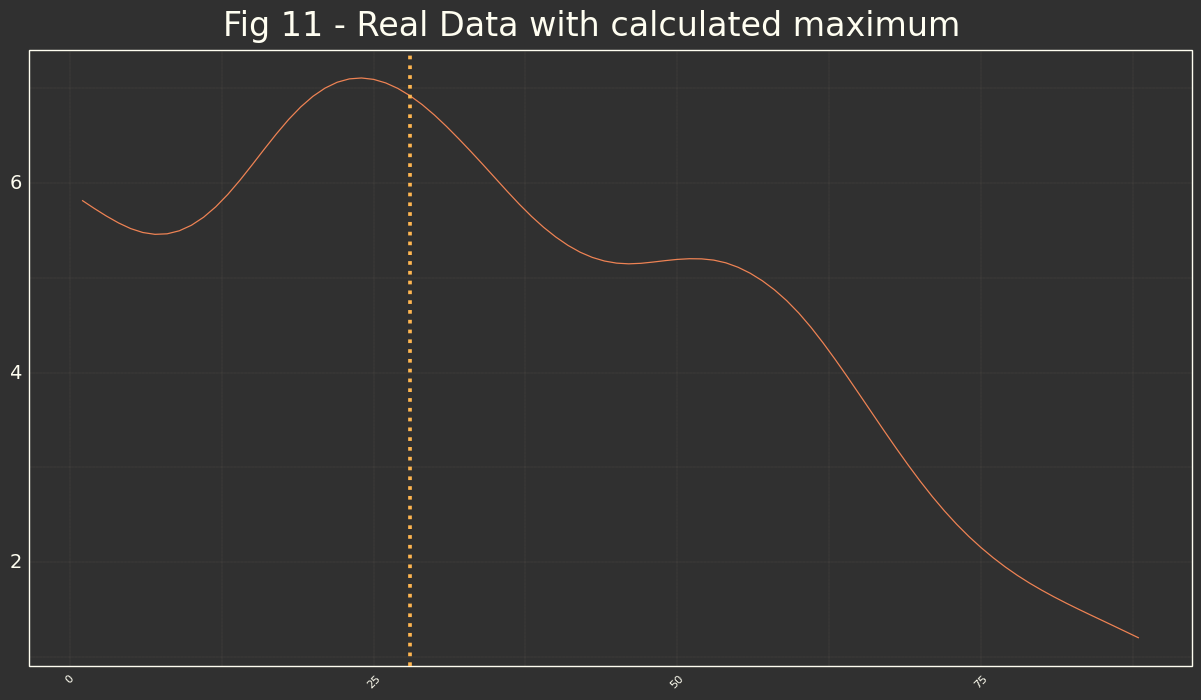It's really near to the real maximum of our curve.

## Quick Check to another Go-Live

Let me avoid some code or some explanations (steps are exactly the same as above) and go to the principal insights of the modelization.

Data Origin We are looking for the same sqlite DB, but for different project.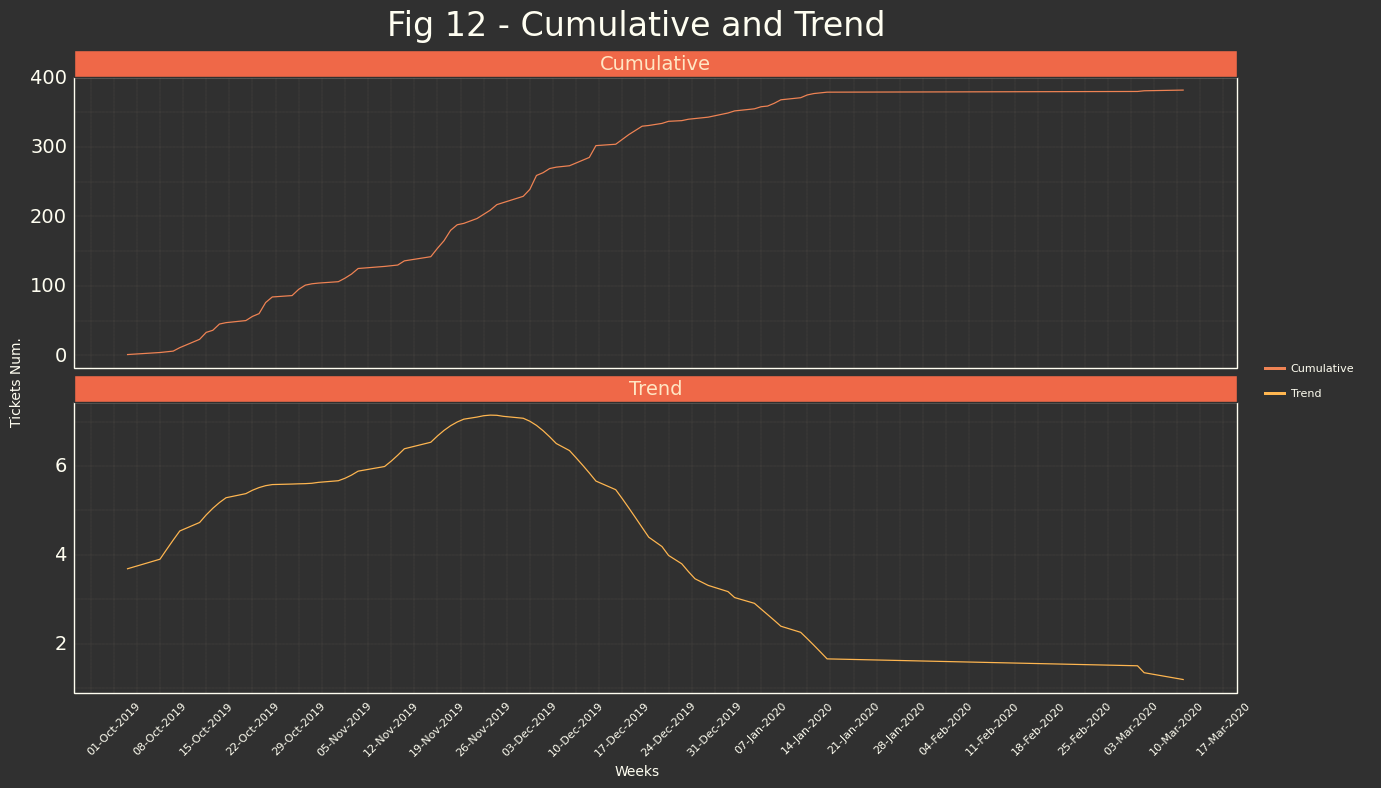### Model and Analysis

Some data for our adjustment:
--------------------------------
Initial number of tickets:  1
Current number of tickets:  382
Number of days:             77

Final parameters for our modeled function:
------------------------------------------
a = 438.471 +/- 6.288
b = 3.633 +/- 0.087
c = 22.655 +/- 0.625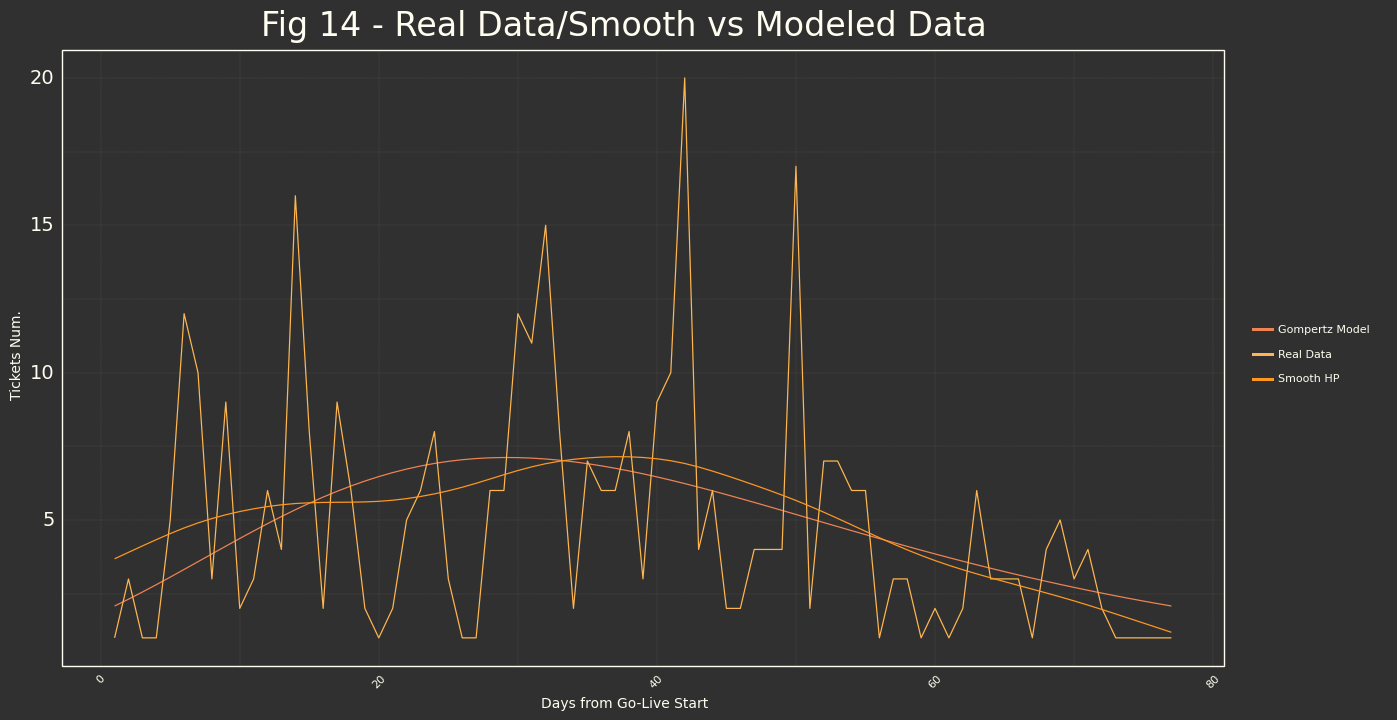Mean squared log error (MSLE):  0.116
Root Mean squared error (RMSE):  7.963
R2 score:  0.996
How zero is our solution? -0.0
Days to the Maximum since Go-Live:  29
Day of flattening of Post Go-Live Support:   01, Nov 2019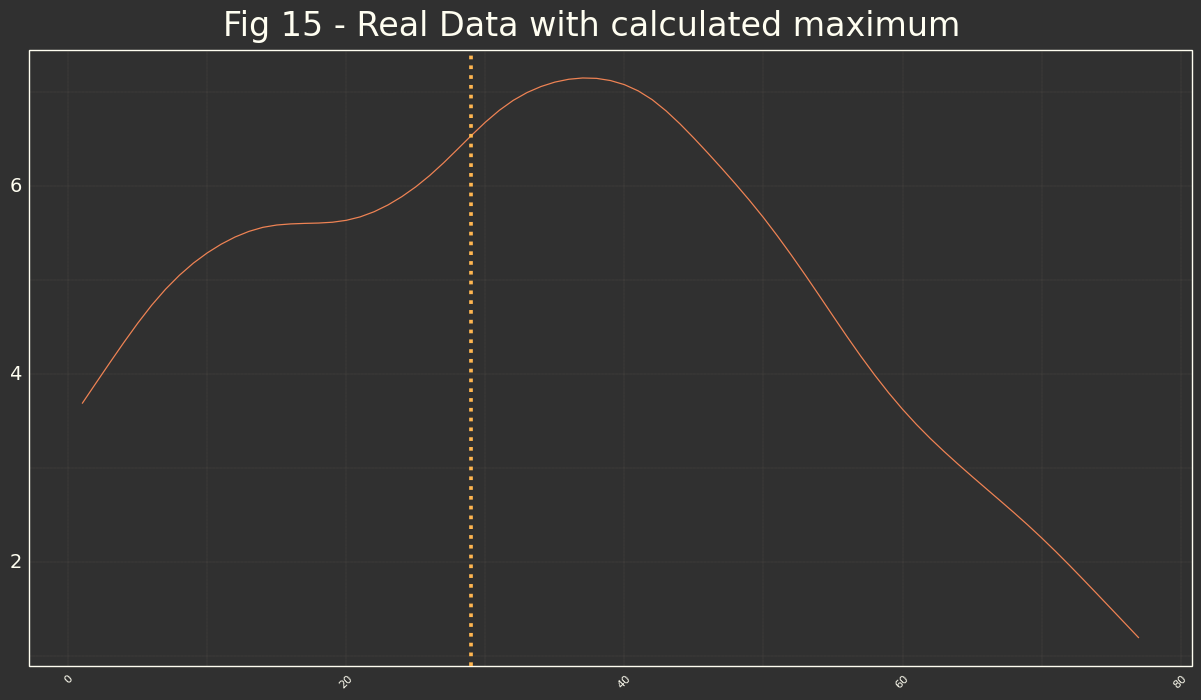## Conclusions

I think that we are quite sure that our hypothesis that Gompertz Function and its study will let us to describe objective way Go-Live process in an IT department has been demonstrated ($R^2 > 0.96$ each time)

With 3 different numbers ($a,b,c$) we are able to know how Go-Live process has been executed, calculate its maximum, etc...

Each IT Department will be the responsible then, that using this 3 parameters and the meaning of all of them to decide which values are 'correct' or intervals of confidence that brings an objective way to stablish some KPI for the Go-Live projects. It could be necessary a bit of work as you need to compare through other projects of your company to be able to know which is the normal situation of a Go-Live project.

For example, using only my 2 samples, it seems that in a Global Go-Live of studied company that impact all the users of the company, go-live and post-golive support should have 1 month of really hard work till the maximum of ticket creation, and then 2 month aprox of stabilization of the new functionality. If this is usual behavior of the global projects, it will let us to standardize some type of start point to begin to improving this numbers for example for future projects, or to escalate support/project teams, etc...

Scope: I am not sure how the model could be useful for real-time analysis or it's only KPI/Metric values to evaluate success of Go-Live process. I think that yes, could it be possible to add alerts if current values project some not desired data (maximums, asymptotic values, etc...)

#python #non-linear #modelization

Library
pandas
numpy
plotnine
sqlite3
scipy
sklearn

### Data Origin

Data Origin
sqlite3 Data Base

### DS Algorithm/Model

Model
Non-Linear Least Squares

### Outputs

Function parameters
Gompertz-Function parameter $a$
Gompertz-Function parameter $b$
Gompertz-Function parameter $c$
Maximum of Tickets Curve
Accuracy Values